# Turtle Graphics

(back to contents)

Turtle draws a plant which is represented as a sentence of words. An L-system is a set of rules and an axiom which produces a sentence that is interpreted by turtle. If no rules are specified (like in this chapter), the axiom itself is the sentence to be interpreted. Words in the sentence are commands for the turtle. Words (commands) can have arguments which may be arbitrary LISP s-expressions.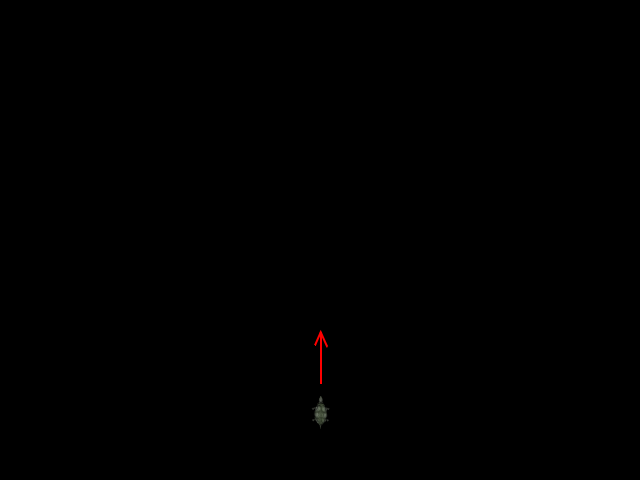Turtle starts its life in the bottom middle of the screen. Initially turtle's nose is pointing up like a space rocket ready for launch.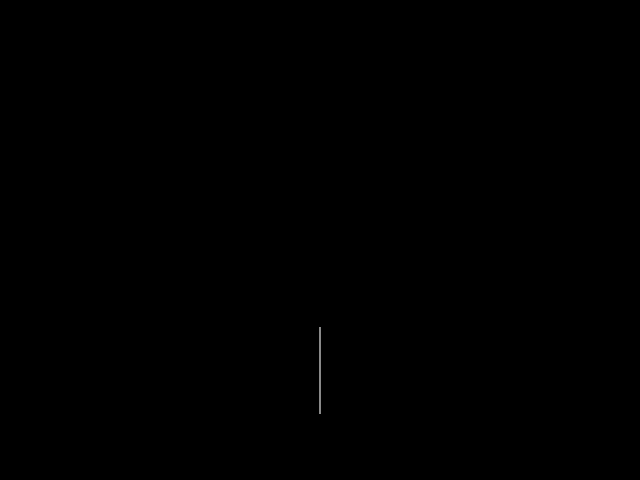```(with-graphics (set-axiom (forward(150)))) ```

Issuing the command `forward(150)` makes turtle go 150 units forward and leave a trace behind. Traces that turtle leaves behind are somewhat like cylindrical water pipes.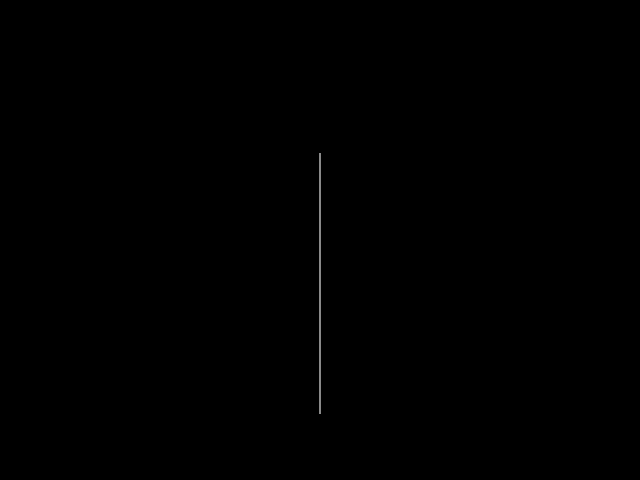```(with-graphics (set-axiom (forward(150) forward(150) forward(150)))) ```

Three times `forward(150)` in a row is essentially the same as once `forward(450)`; it will make turtle leave a longer trace than in the previous example. There is an abbreviation for "go forward" command — the letter `F`. If used without argument, it makes turtle go forward 10 units.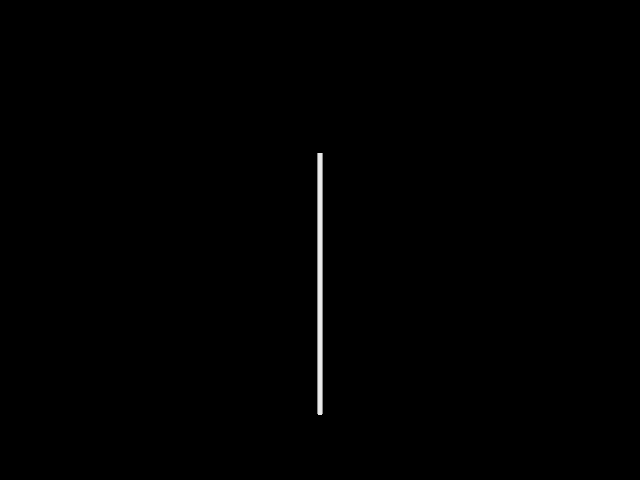```(with-graphics (set-axiom (radius(5) forward(150) forward(150) forward(150)))) ```

Another word that turtle understands is `radius`. It changes the radius (thickness) of trace left behind by turtle.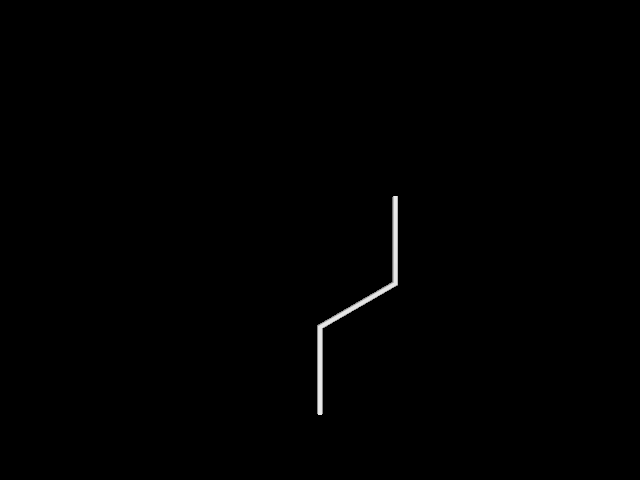```(with-graphics (set-axiom (radius(5) forward(150) turn(60) forward(150) turn(-60) forward(150)))) ```

Turtle can change its direction. One of the commands that make turtle change direction is `turn`. It takes angle in degrees as argument and rotates turtle left or right, depending on whether angle is negative or positive.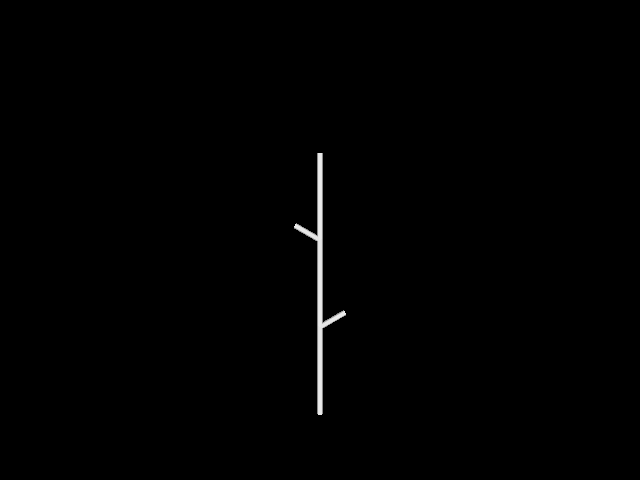```(with-graphics (set-axiom (radius(5) forward(150) [ turn(60) forward(50) ] forward(150) [ turn(-60) forward(50) ] forward(150)))) ```

Two of the most fundamental turtle commands for plant drawing are `[` and `]` (left square bracket and right square bracket). `[` makes turtle remember its current position, orientation, color and radius. More precisely, `[` pushes turtle's state onto a stack. The command `]` makes turtle go back to its last remembered position and restore orientation, color and radius. The `]` command pops turtle's previous state off the stack.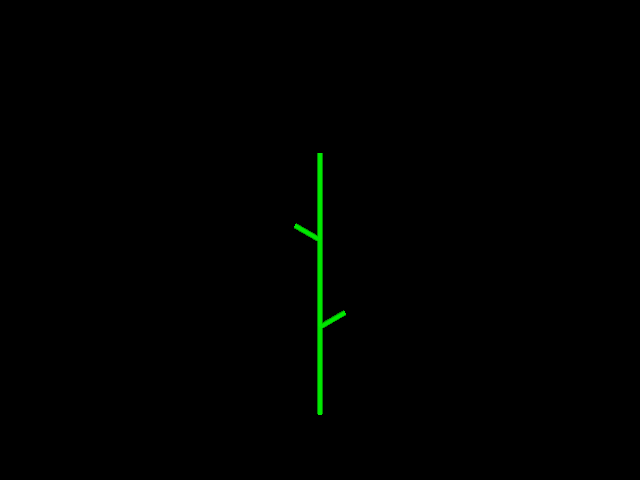```(with-graphics (set-axiom (radius(5) color((create-color 0.0 1.0 0.0)) forward(150) [ turn(60) forward(50) ] forward(150) [ turn(-60) forward(50) ] forward(150)))) ```

To change turtle's color, issue the command `color`. This command takes color data structure as argument. Color data structure can be created with lisp function `create-color` which takes three arguments: red, green and blue color component intensity values represented as numbers from 0.0 to 1.0.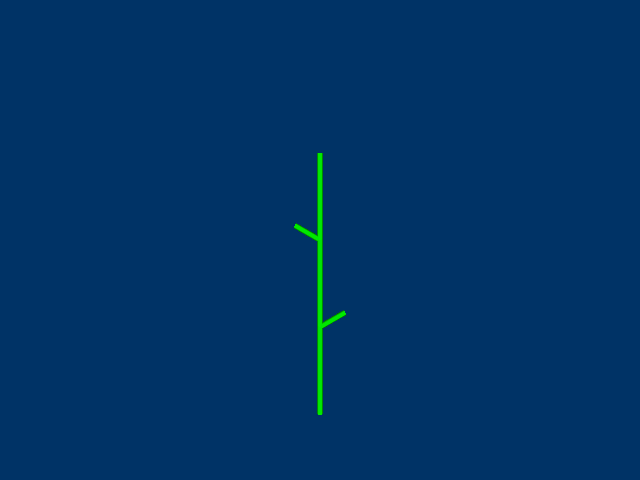```(with-graphics (set-background-color (create-color 0.0 0.2 0.4)) (set-axiom (radius(5) color((create-color 0.0 1.0 0.0)) forward(150) [ turn(60) forward(50) ] forward(150) [ turn(-60) forward(50) ] forward(150)))) ```

`set-background-color` is not a turtle command. It is just a function that configures turtle's environment. It takes color data structure as argument.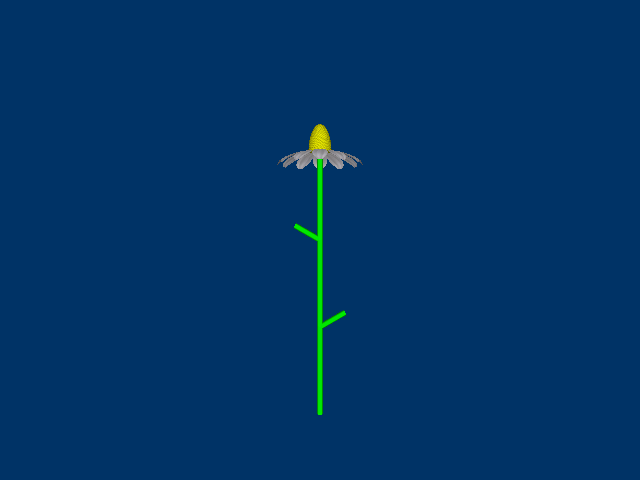```(with-graphics (read-wavefront "blossom.obj" "blossom.png") (set-background-color (create-color 0.0 0.2 0.4)) (set-axiom (radius(5) color((create-color 0.0 1.0 0.0)) forward(150) [ turn(60) forward(50) ] forward(150) [ turn(-60) forward(50) ] forward(150) obj("blossom" 20.0)))) ```

Turtle can do more than move around and leave traces. Turtle can also drop predefined 3D mesh objects. Mesh objects can be created with 3D modeling software (e.g., blender) and then exported as wavefront object files. To load such files, the function `read-wavefront` must be called. `read-wavefront` takes two arguments: wavefront object file name and texture file name. The texture file must be in portable network graphics (PNG) format. Texture file name argument is optional. Once wavefront object file is loaded, turtle can reference objects by their name. Turtle command that drops objects is `obj`. The `obj` command can take one, two or three arguments. First argument is the name of the object to drop. Second argument is the amount of scaling to apply to the object before dropping. Third argument is the color of the object, which can be used in case texture has not been specified.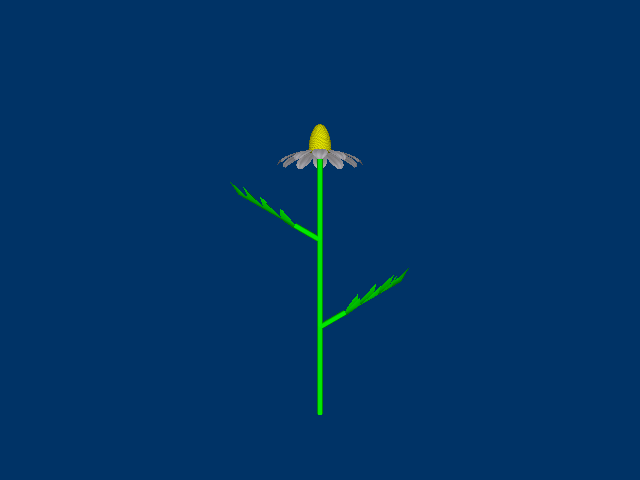```(defparameter *green* (create-color 0.0 1.0 0.0)) (with-graphics (read-wavefront "leaf.obj") (read-wavefront "blossom.obj" "blossom.png") (set-background-color (create-color 0.0 0.2 0.4)) (set-axiom (radius(5) color(*green*) forward(150) [ turn(60) forward(50) obj("leaf" 20.0 *green*) ] forward(150) [ turn(-60) forward(50) roll(180) obj("leaf" 20.0 *green*) ] forward(150) obj("blossom" 20.0)))) ```

Notice that before dropping the second leaf object, `roll(180)` command is issued. If turtle does not roll there, then the second leaf is drawn upside down. Commands `turn`, `pitch` and `roll` are fundamental for turtle rotation. Animations below illustrate how they work.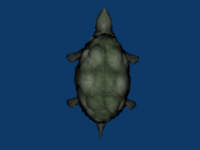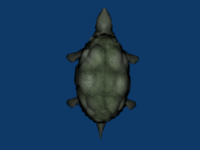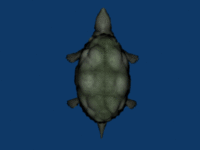turn pitch roll

(back to contents)